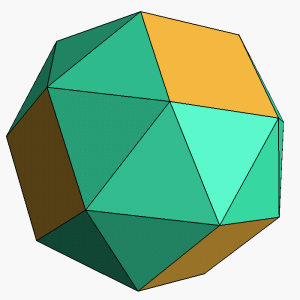# Peculiar Cube

Geometry Level 4A convex, vertex-transitive polyhedron is composed of squares and equilateral triangles with 5 faces joining at each vertex.

If $x$ is the number of square faces and $y$ is the number of triangle faces, compute $xy.$

×